JEE  >  JEE Main Previous year questions (2016-20): Ray & Wave Optics- 2

# JEE Main Previous year questions (2016-20): Ray & Wave Optics- 2 - Notes | Study Physics For JEE - JEE

 1 Crore+ students have signed up on EduRev. Have you?

Q 40. A planoconvex lens becomes an optical system of 28 cm focal length when its plane surface is silvered and illuminated from left to right as shown in Fig-A.
If the same lens is instead silvered on the curved surface and illuminated from other side as in Fig. B, it acts like an optical system of focal length 10 cm.

The refractive index of the material of lens is: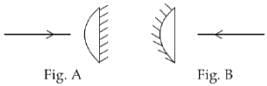(1) 1.51
(2) 1.75
(3) 1.50
(4) 1.55
Ans:
D
Solution:
When plane surface is silvered
Focal length f1 =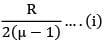and f1 = 28 cm
When curved surface is silvered
Focal length f2 = R/2μ ... (ii)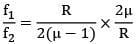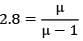µ = 1.55

Q 41. Unpolarized light of intensity I is incident on a system of two polarizers, A followed by B. The intensity of emergent light is I/2. If a third polarizer C is placed between A and B the intensity of emergent light is reduced to I/3. The angle between the polarizers A and C is θ, then     
(1)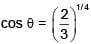(2)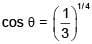(3)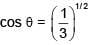(4)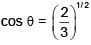Ans:
A
Solution:
A and B have same alignment of transmission axis.
Lets assume c is introduced at θ angle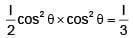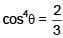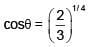Q 42. A ray of light is incident at an angle of 60° on one face of a prism of angle 30°. The emergent ray of light makes an angle of 30° with incident ray. The angle made by the emergent ray with second face of prism will be:    
(1) 0°
(2) 90°
(3) 30°
(4) 45°
Ans:
B
Solution:
δ = I + e - A
30 = 60 + e – 30°
⇒ e = 0
So angle with face = 90°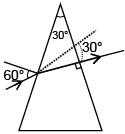Q 43. A diverging lens with magnitude of focal length 25 cm is placed at a distance of 15 cm from a converging lens of magnitude of focal length 20 cm. A beam of parallel light falls on the diverging lens. The final image formed is    (2017)
(1) Real and at a distance of 40 cm from the divergent lens
(2) Real and at a distance of 6 cm from the convergent lens
(3) Real and at a distance of 40 cm from convergent lens

(4) Virtual and at a distance of 40 cm from convergent lens
Ans:
(3)
Solution: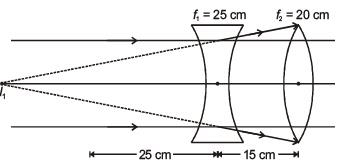For converging lens, u = –40 cm which is equal to 2f
∴ Image will be real and at a distance of 40 cm from convergent lens.

Q 44. In a Young's double slit experiment, the slits are separated by 0.5 mm, and the screen is placed 150 cm away. A beam of light consisting of two wavelengths, 650 nm and 520 nm, is used to obtain interference fringes on the screen. The least distance from the common central maximum to the point where the bright fringes due to both the wavelengths coincide is    (2017)
(1) 9.75 mm
(2) 15.6 mm
(3) 1.56 mm
(4) 7.8 mm
Ans:
(4)
Solution: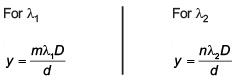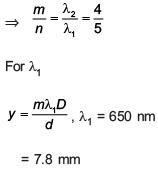Q 45.  A diverging lens with magnitude of focal length 25 cm is placed at a distance of 15 cm from a converging lens of magnitude of focal length 20 cm. A beam of parallel light falls on the diverging lens. The final image formed is    
(1) Real and at a distance of 40 cm from the divergent lens
(2) Real and at a distance of 6 cm from the convergent lens
(3) Real and at a distance of 40 cm from convergent lens
(4) Virtual and at a distance of 40 cm from convergent lens
Ans:
(3)
Solution:For converging lensu = –40 cm which is equal to 2f
∴ Image will be real and at a distance of 40 cm from convergent lens.

Q 46.  In a Young's double slit experiment, slit s are separated by 0.5 mm, and the screen is placed 150 cm away. A beam of light consisting of two wavelengths, 650 nm and 520 nm, is used to obtain interference fringes on the screen. The least distance from the common central maximum to the point where the bright fringes due to both the wavelengths coincide is    
(1) 9.75 mm
(2) 15.6 mm
(3) 1.56 mm
(4) 7.8 mm
Ans:
(4)
Solution: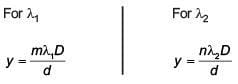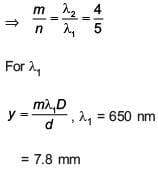Q 47. The maximum velocity of the photoelectrons emitted from the surface is v when light of frequency n falls on a metal surface. If the incident frequency is increased to 3n, the maximum velocity of the ejected photoelectrons will be:   
(1) less than √3 v
(2) v
(3) more than √3 v
(4) equal to √3 v
Ans:
(4)
Solution: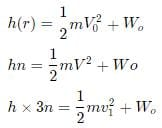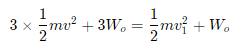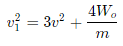solving the above equation, we get
v1 = √3 v

Q 48. An object is dropped from a height h from the ground. Every time it hits the ground it looses 50% of its kinetic energy. The total distance covered as t→∞ is:    
(1) 3h
(2) ∞
(3) 5/3h
(4)  8/3h
Ans:
(1)
Solution: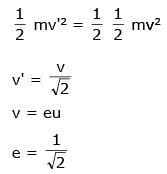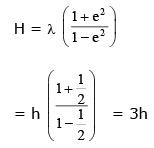Q 49.  N moles of a diatomic gas in a cylinder are at a temperature T. Heat is supplied to the cylinder such that the temperature remains constant but n moles of the diatomic gas get converted into monoatomic gas. What is the change in the total kinetic energy of the gas?    
(1) 0
(2)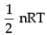(3)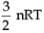(4)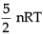Ans:
B
Solution: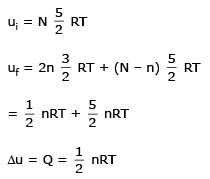Q 50.  For the P-V diagram given for an ideal gas,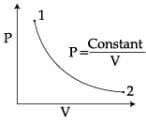out of the following which one correctly represents the T-P diagram?    
A: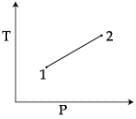B: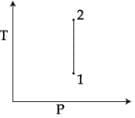C: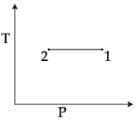D: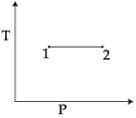Ans:
(3)
Solution: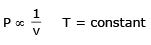Q 51. An observer looks at a distant tree of height 10 m with a telescope of magnifying power 20. To the observer the tree appears :    
(1) 10 times taller
(2) 10 times nearer
(3) 20 times taller
(4) 20 times nearer
Ans:
(4)
Solution:
Length of Astronomical Telescope -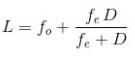- Wherein
f0 = Focal length of objective
fe = focal length of eyepiece
Telescope resolves and brings the object closer which is far away from the telescope. Hence for telescope with magnifying power 20, the tree appears  20 times nearer.
Correct option is 4.

Q 52. The box of a pin hole camera, of length L, has a hole of radius a. It is assumed that when the hole is illuminated by a parallel beam of light of wavelength λ the spread of the spot (obtained on the opposite wall of the camera) is the sum of its geometrical spread and the spread due to diffraction. The spot would then have its minimum size (say bmin) when:    
(1)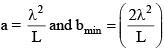(2)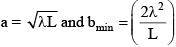(3)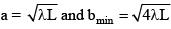(4)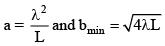Ans:
(3)

Solution: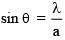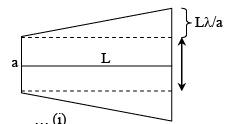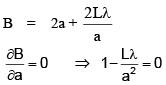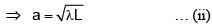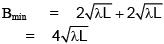[By substituting for a from (ii) in (i)]
∴ The radius of the spot =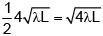Q 53. In an experiment for determination of refractive index of glass of a prism by i - δ, plot, it was found that a ray incident at angle 35°, suffers a deviation of 40° and that it emerges at angle 79°. In that case which of the following is closest to the maximum possible value of the refractive index?    
(1) 1.5
(2) 1.6
(3) 1.7
(4) 1.8
Ans:
(1)
Solution: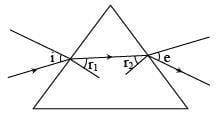δ =  i + e - A
40 = 35 + 79 - A
40 = 114 - A
A = 114 - 40 = 74 = r1+ r2
From this we get,
μ = 1.5
∴ δmin < 40°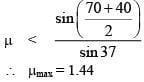Q 54. In Young's double slit experiment, the distance between slits and the screen is 1.0 m and monochromatic light of 600 nm is being used. A person standing near the slits is looking at the fringe pattern . When the separation between the slits is varied, the interference pattern disappears for a particular distance d0 between the slits. If the angular resolution of the eye is 10/60 the value of d0 is close to    
(1) 2 mm
(2) 1 mm
(3) 3 mm
(4) 4 mm

Ans: (1)
Solution:
Angular fringe width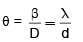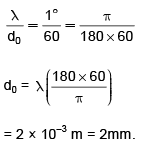Q 55. To find the focal length of a convex mirror, a student records the following data: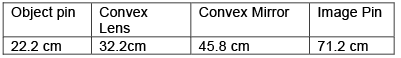The focal length of the convex lens is f1 and that of mirror is f2. Then taking index correction to be negligibly small, f1 and f2 are close to:    
(1) f1 = 15.6 cm f2 = 25.4 cm
(2) f1 = 7.8 cm f2 = 12.7 cm
(3) f1 = 7.8 cm f2 = 25.4 cm
(4) f1 = 12.7 cm f2 = 7.8 cm
Ans:
(2)
Solution:
For convex lens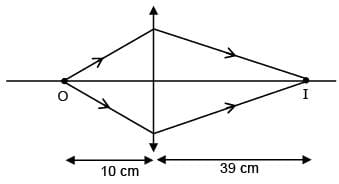u =–10 cm
v = 39 cm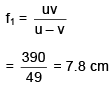For convex mirror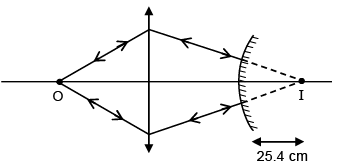R = 25.4 cm
f2 = 12.7 cm

Q 56. A convex lens, of focal length 30 cm, a concave lens of focal length 120 cm, and a plane mirror are arranged as shown. For an object kept at a distance of 60 cm from the convex lens, the final image, formed by the combination, is a real image, at a distance of: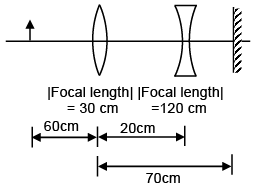(1) 70 cm from the concave lens
(2) 60 cm from the convex lens
(3) 60 cm from the concave lens
(4) 70 cm from the convex lens
Ans:
(Bonus)
Solution: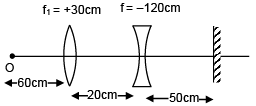Event 1 - Refraction from convex lens.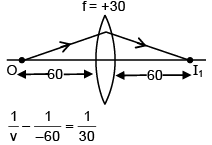v = +60 cm
Event 2 - Refraction from concave lens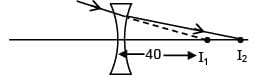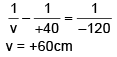Event 3 - Refraction from plane mirror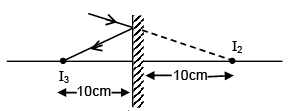Event 4 - Refraction from concave lens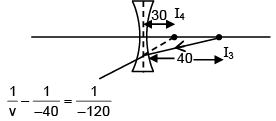v = –30
Event 5 - Refraction from convex lens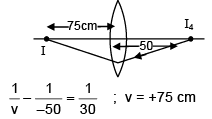Q 57. To determine refractive index of glass slab using a travelling microscope, minimum number of readings required are:     
(1) Four
(2) Two
(3) Three
(4) Five
Ans: (3)
Solution.

Q 58. A hemispherical glass body of radius 10 cm and refractive index 1.5 is silvered on its curved surface. A small air bubble is 6 cm below the flat surface inside it along the axis. The position of the image of the air bubble made by the mirror is seen.: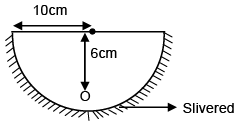(1) 20 cm below flat surface
(2) 30 cm below flat surface
(3) 16 cm below flat surface
(4) 14 cm below flat surface

Ans: (1)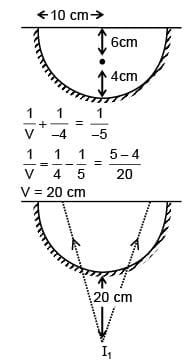I1 acts as object for plane surface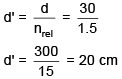20 cm below plane surface

Q 59. Two stars are 10 light years away from the earth. They are seen through a telescope of objective diameter 30 cm. The wavelength of light 600 nm. To see the stars just resolved by the telescope, the minimum distance between them should be (1 light year = 9.46 × 1015 m) of the order of:    
(1) 108 km
(2) 106 km
(3) 1011 km
(4) 1010 km
Ans:
(2)
Solution: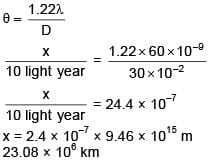The document JEE Main Previous year questions (2016-20): Ray & Wave Optics- 2 - Notes | Study Physics For JEE - JEE is a part of the JEE Course Physics For JEE.
All you need of JEE at this link: JEE

## Physics For JEE

258 videos|633 docs|256 tests

## Physics For JEE

258 videos|633 docs|256 tests

Track your progress, build streaks, highlight & save important lessons and more!

,

,

,

,

,

,

,

,

,

,

,

,

,

,

,

,

,

,

,

,

,

;